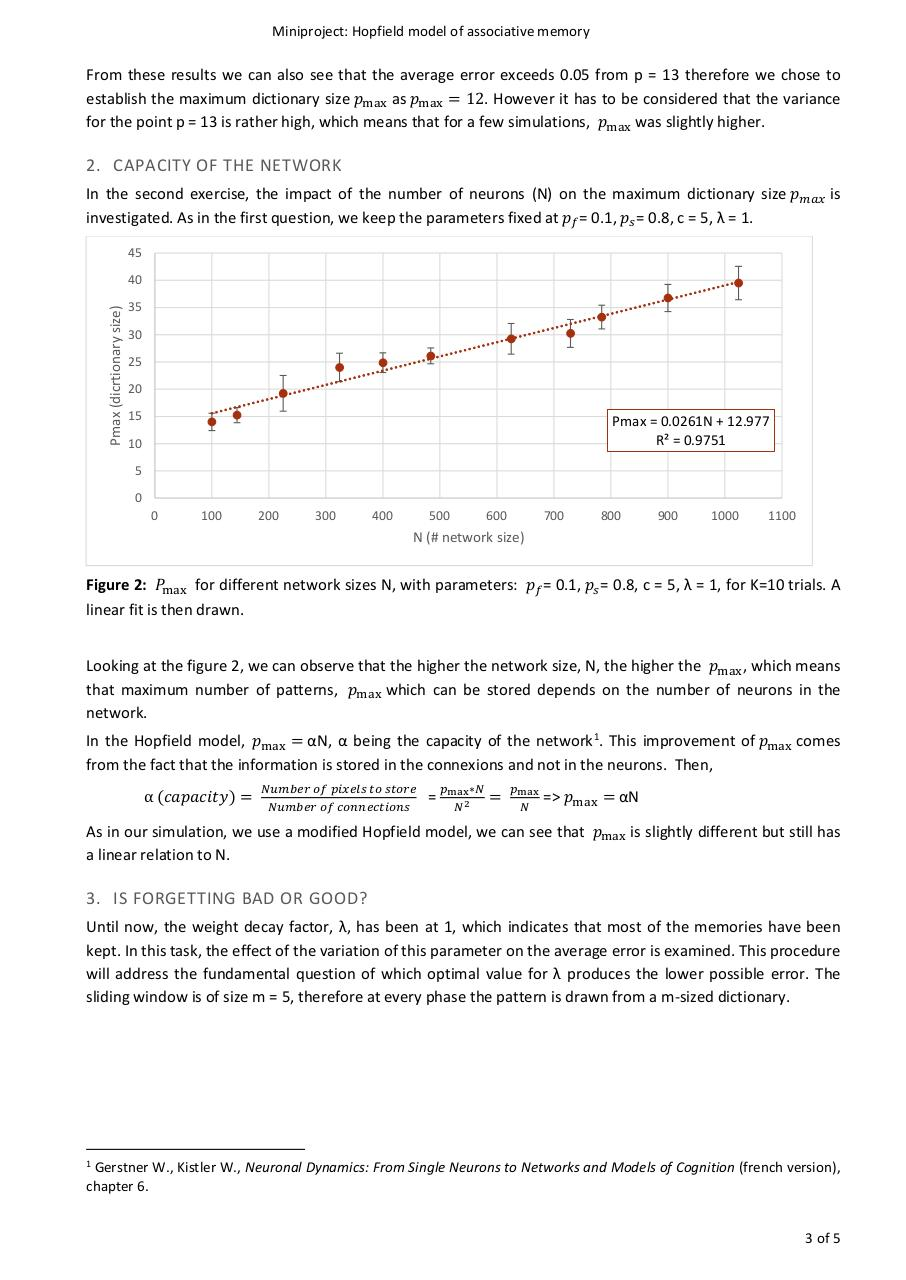# Projet Hopfield.pdfPage 1 2 3 4 5 6

#### Text preview

Miniproject: Hopfield model of associative memory

From these results we can also see that the average error exceeds 0.05 from p = 13 therefore we chose to
establish the maximum dictionary size 𝑝𝑝max as 𝑝𝑝max = 12. However it has to be considered that the variance
for the point p = 13 is rather high, which means that for a few simulations, 𝑝𝑝max was slightly higher.

2. CAPACITY OF THE NETWORK

In the second exercise, the impact of the number of neurons (N) on the maximum dictionary size 𝑝𝑝𝑚𝑚𝑚𝑚𝑚𝑚 is
investigated. As in the first question, we keep the parameters fixed at 𝑝𝑝𝑓𝑓 = 0.1, 𝑝𝑝𝑠𝑠 = 0.8, c = 5, λ = 1.
45

Pmax (dicrtionary size)

40
35
30
25
20
15

Pmax = 0.0261N + 12.977
R² = 0.9751

10
5
0
0

100

200

300

400

500

600

700

800

900

1000

1100

N (# network size)

Figure 2: 𝑃𝑃max for different network sizes N, with parameters: 𝑝𝑝𝑓𝑓 = 0.1, 𝑝𝑝𝑠𝑠 = 0.8, c = 5, λ = 1, for K=10 trials. A
linear fit is then drawn.
Looking at the figure 2, we can observe that the higher the network size, N, the higher the 𝑝𝑝max , which means
that maximum number of patterns, 𝑝𝑝max which can be stored depends on the number of neurons in the
network.

In the Hopfield model, 𝑝𝑝max = αN, α being the capacity of the network 1. This improvement of 𝑝𝑝max comes
from the fact that the information is stored in the connexions and not in the neurons. Then,
α (𝑐𝑐𝑐𝑐𝑐𝑐𝑐𝑐𝑐𝑐𝑐𝑐𝑐𝑐𝑐𝑐) =

𝑁𝑁𝑁𝑁𝑁𝑁𝑁𝑁𝑁𝑁𝑁𝑁 𝑜𝑜𝑜𝑜 𝑝𝑝𝑝𝑝𝑝𝑝𝑝𝑝𝑝𝑝𝑝𝑝 𝑡𝑡𝑡𝑡 𝑠𝑠𝑠𝑠𝑠𝑠𝑠𝑠𝑠𝑠
𝑁𝑁𝑁𝑁𝑁𝑁𝑁𝑁𝑁𝑁𝑁𝑁 𝑜𝑜𝑜𝑜 𝑐𝑐𝑐𝑐𝑐𝑐𝑐𝑐𝑐𝑐𝑐𝑐𝑐𝑐𝑐𝑐𝑐𝑐𝑐𝑐𝑐𝑐

=

𝑝𝑝max ∗𝑁𝑁
𝑁𝑁 2

=

𝑝𝑝max
𝑁𝑁

=&gt; 𝑝𝑝max = αN

As in our simulation, we use a modified Hopfield model, we can see that 𝑝𝑝max is slightly different but still has
a linear relation to N.

3. IS FORGETTING BAD OR GOOD?
Until now, the weight decay factor, λ, has been at 1, which indicates that most of the memories have been
kept. In this task, the effect of the variation of this parameter on the average error is examined. This procedure
will address the fundamental question of which optimal value for λ produces the lower possible error. The
sliding window is of size m = 5, therefore at every phase the pattern is drawn from a m-sized dictionary.

Gerstner W., Kistler W., Neuronal Dynamics: From Single Neurons to Networks and Models of Cognition (french version),
chapter 6.

1

3 of 5